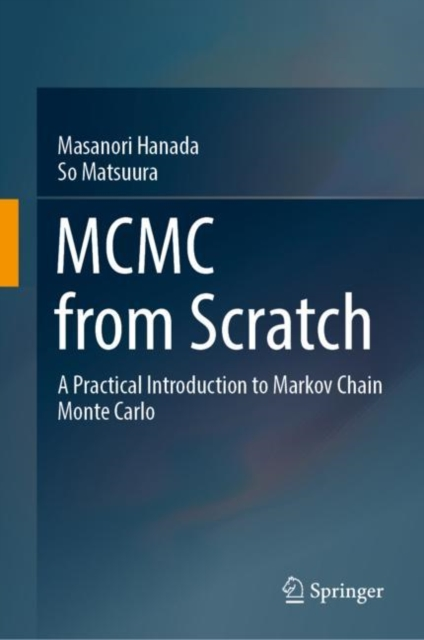# MCMC from Scratch : A Practical Introduction to Markov Chain Monte Carlo Hardback

Hardback

• Information

#### Description

This textbook explains the fundamentals of Markov Chain Monte Carlo (MCMC) without assuming advanced knowledge of mathematics and programming.

MCMC is a powerful technique that can be used to integrate complicated functions or to handle complicated probability distributions.

MCMC is frequently used in diverse fields where statistical methods are important - e.g.

Bayesian statistics, quantum physics, machine learning, computer science, computational biology, and mathematical economics.

This book aims to equip readers with a sound understanding of MCMC and enable them to write simulation codes by themselves. The content consists of six chapters. Following Chap. 2, which introduces readers to the Monte Carlo algorithm and highlights the advantages of MCMC, Chap. 3 presents the general aspects of MCMC. Chap. 4 illustrates the essence of MCMC through the simple example of the Metropolis algorithm.

In turn, Chap. 5 explains the HMC algorithm, Gibbs sampling algorithm and Metropolis-Hastings algorithm, discussing their pros, cons and pitfalls.

Lastly, Chap. 6 presents several applications of MCMC. Including a wealth of examples and exercises with solutions, as well as sample codes and further math topics in the Appendix, this book offers a valuable asset for students and beginners in various fields.

£44.99

£33.36